• Blog

# Curiosity-Driven Learning Through Next State Prediction

Tech Blog Thomas Simonini

In the last few years, we’ve seen a lot of breakthroughs in reinforcement learning (RL). From 2013 with the first deep learning model to successfully learn a policy directly from pixel input using reinforcement learning to the OpenAI Dexterity project in 2019, we live in an exciting moment in RL research.

Today, we’ll learn about curiosity-driven learning methods, one of the most promising series of strategies in deep reinforcement learning. Thanks to this method, we were able to successfully train an agent that wins the first level of Super Mario Bros with only curiosity as a reward.

Remember that RL is based on the reward hypothesis, which is the idea that each goal can be described as the maximization of the rewards. However, the current problem with extrinsic rewards (i.e., rewards given by the environment) is that this function is hard coded by a human, which is not scalable to real world problems (such as designing a good reward function for autonomous vehicles).

The idea of curiosity-driven learning is to build a reward function that is intrinsic to the agent (generated by the agent itself). In this sense, the agent will act as a self-learner since it will be the student, but also its own feedback master.

Sound crazy? Yes, probably. That’s the genius idea that was reintroduced in the 2017 paper Curiosity-Driven Exploration by Self-Supervised Prediction. The results were then improved with the second paper Large-Scale Study of Curiosity-Driven Learning. Why reintroduced? Because curiosity has been a subject of research in RL since the 90s with the amazing work of Mr. J. Schmidhuber, which you can read here.

They discovered that curiosity-driven learning agents perform as well as if they had extrinsic rewards and that they were able to generalize better with unexplored environments.

## Two Major Problems in Modern RL

Modern RL suffers from two core problems:

First, the sparse rewards or non-existing rewards problem: that is, most rewards do not contain information, and hence are set to zero. However, as rewards act as feedback for RL agents, if they don’t receive any, their knowledge of which action is appropriate (or not) cannot change.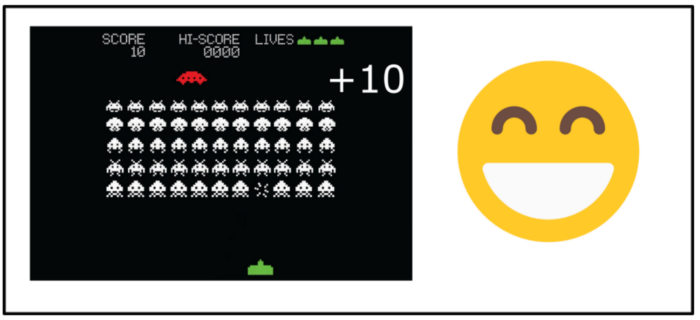Thanks to the reward, our agent knows that this action at that state was good.

For instance, in Vizdoom “DoomMyWayHome,” your agent is only rewarded if it finds the vest. However, the vest is far away from your starting point, so most of your rewards will be zero.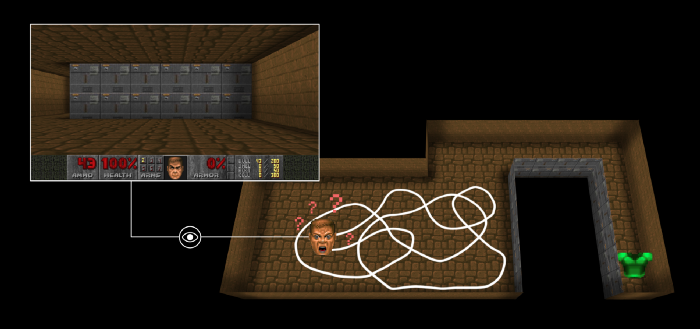Image source: Felix Steger

Therefore, if our agent does not receive useful feedback (dense rewards), it will take much longer to learn an optimal policy.

The second big problem is that the extrinsic reward function is handmade — that is, in each environment, a human has to implement a reward function. But how can we scale that in big and complex environments?

So What Is Curiosity?

Therefore, a solution to these problems is to develop a reward function that is intrinsic to the agent, i.e., generated by the agent itself. This intrinsic reward mechanism is known as curiosity because it explores states that are novel/unfamiliar. In order to achieve that, our agent will receive a high reward when exploring new trajectories.

This reward design is based on how human plays — some suppose we have an intrinsic desire to explore environments and discover new things! There are different ways to calculate this intrinsic reward, and we’ll focus on curiosity through next-state prediction.

## Curiosity Through Prediction-Based Surprise (or Next State Prediction)

Curiosity is an intrinsic reward that is equal to the error of our agent of predicting the next state, given the current state and action taken. More formally, we can define this as: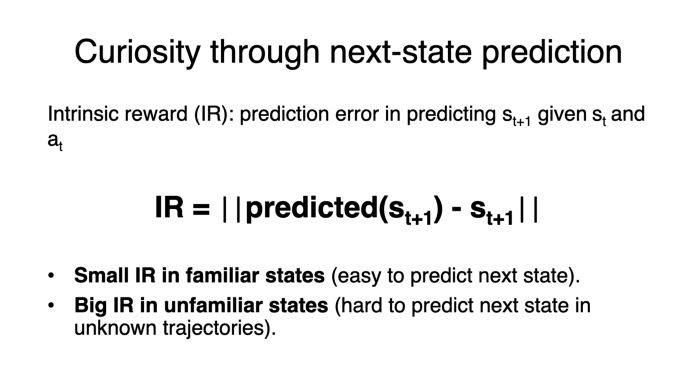Why? Because the idea of curiosity is to encourage our agent to perform actions that reduce the uncertainty in the agent’s ability to predict the consequences of its own actions (uncertainty will be higher in areas where the agent has spent less time, or in areas with complex dynamics).

Let’s break it down further. Say you play Super Mario Bros:

• If you spend a lot of time in the beginning of the game (which is not new), the agent will be able to accurately predict what the next state will be, so the reward will be low.
• On the other hand, if you discover a new room, our agent will be very bad at predicting the next state, so the agent will be pushed to explore this room.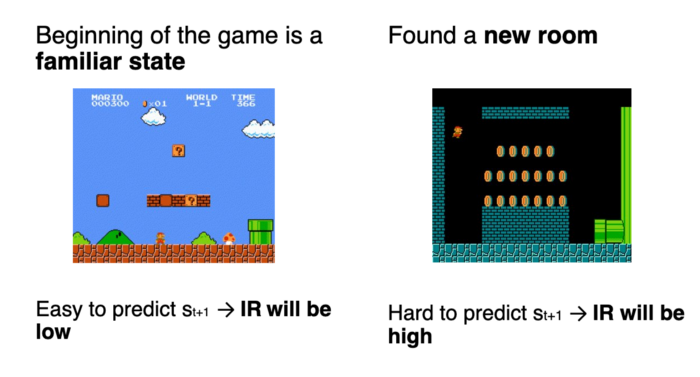Consequently, measuring error requires building a model of environmental dynamics that predicts the next state given the current state and the action. The question that we can ask here is: how can we calculate this error? To calculate curiosity, we will use a module introduced in the first paper called Intrinsic Curiosity module.

## The Need for a Good Feature Space

Before diving into the description of the module, we must ask ourselves: how can our agent predict the next state given our current state and our action?

But we can’t predict s(t+1) by predicting the next frame as we usually do. Why?

First, because it’s hard to build a model that is able to predict high-dimension continuous state space, such as an image. It’s hard to predict the pixels directly, but now imagine you’re in Doom, and you move left — you need to predict 248*248 = 61504 pixels!

It means that in order to generate curiosity, we can’t predict the pixels directly and need to use a better feature representation by projecting the raw pixels space into a feature space that will hopefully only keep the relevant information elements that can be leveraged by our agent.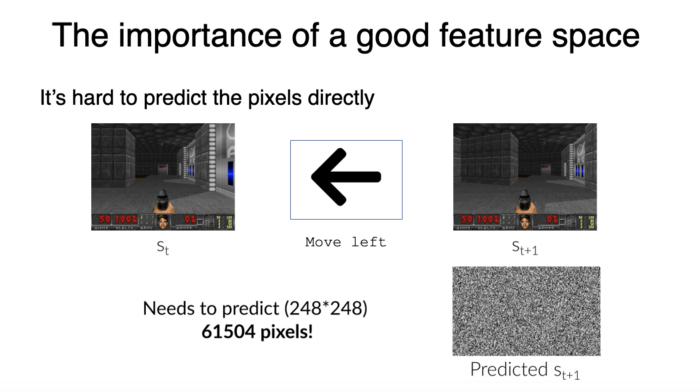Three rules are defined in the original paper for a good feature space representation:

1. Model things that can be controlled by the agent.

2. Model things that can’t be controlled by the agent but can affect the agent.

3. Don’t model things that are not controlled by the agent and have no effect on it.

The second reason we can’t predict s(t+1) by predicting the next frame as we usually do? It might just not be the right thing to do. Imagine you need to study the movement of tree leaves in a breeze. First of all, it’s already hard enough to model the breeze, so predicting the pixel location of each leaf at each time step is even harder.

Instead of making predictions in the raw sensory space (pixels), we need to transform the raw sensory input (array of pixels) into a feature space with only relevant information.

Let’s take this example: your agent is a self driving car. If we want to create a good feature representation, we need to model the below: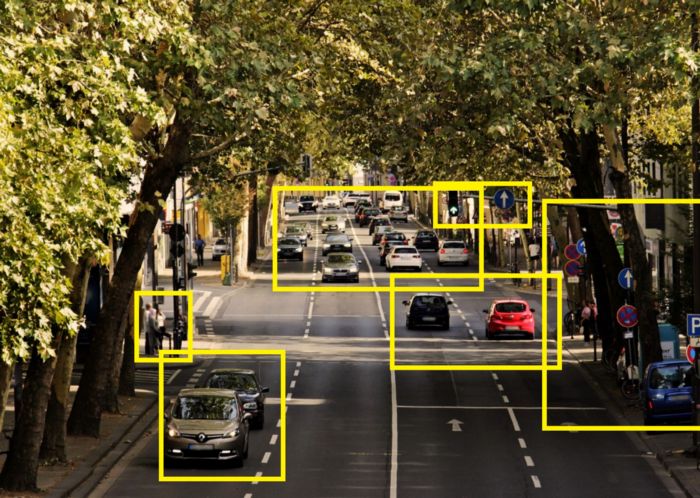The yellow boxes are the important elements.

A good feature representation would be our car (controlled by our agent) and the other cars (we can’t control it, but that can affect the agent), but we don’t need to model the leaves (it doesn’t affect the agent, and we can’t control it). By only keeping this information, we will have a feature representation with less noise.

The desired embedding space should:

• Be compact in terms of dimensional (remove irrelevant parts of the observation space).
• Preserve sufficient information about the observation.
• Be stable, because non-stationary rewards (rewards that decrease through time since curiosity decreases through time) make it difficult for reinforcement agents to learn.

In order to calculate the predicted next state and the real feature representation of the next state, we can use an Intrinsic Curiosity Module (ICM).

## The ICM Module

The ICM Module is the system that helps us to generate curiosity. It’s composed of two neural networks; each of them has an important task.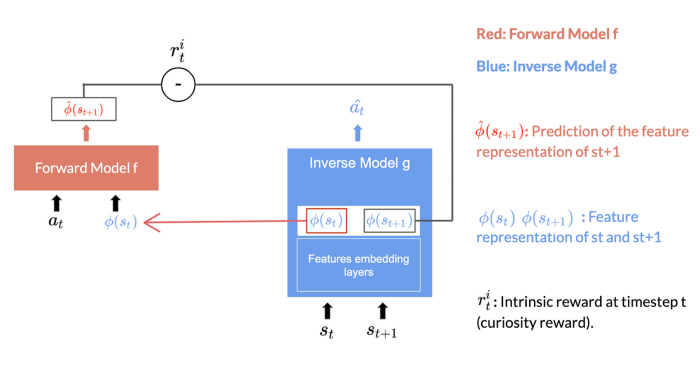The Inverse Model That Generates the Feature Representation of State and Next State

The Inverse Model (g), aims at predicting the action â(t), and in doing so, it learns an internal feature representation of the state and next state, denoted by Φ(s(t)) and Φ(s(t+1)).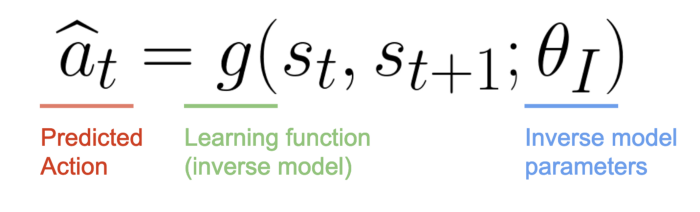You may ask, why do we need to predict the action if we want Φ(s(t)) and Φ(s(t+1))? Remember that we want our state representations to only consider elements that can affect our agent or that it can control.

Since this neural network is only used to predict the action, it has no incentive to represent within its feature embedding space the factors of variation in an environment that does not affect the agent itself.

Lastly, the inverse model loss is given by the cross entropy between our predicted action â(t) and the real action a(t):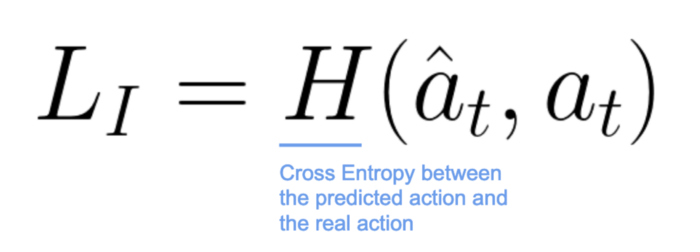The Forward Model That Predicts the Feature Representation of the Next State

The Forward Model (f), predicts the feature representation of the next state hat_Φ(s(t+1)) given Φ(s(t)) and a(t).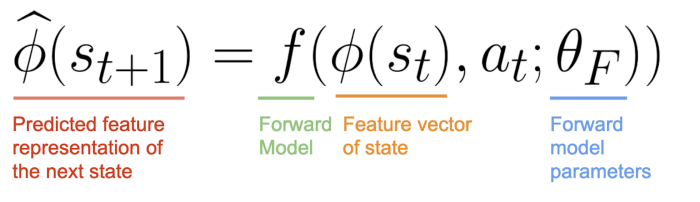The forward model takes as input Φ(s(t)) and at and predict the feature representation Φ(s(t+1)) of s(t+1)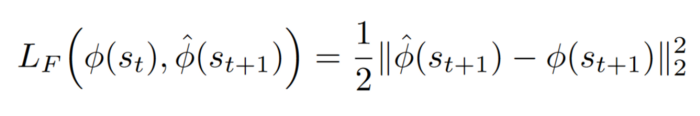Consequently, curiosity will be a real number, i.e., the L2 normed difference between our predicted feature vector of the next state hat_Φ(s(t+1)) (predicted by the Forward Model) and the real feature vector of the next state Φ(s(t+1)) (generated by the Inverse Model).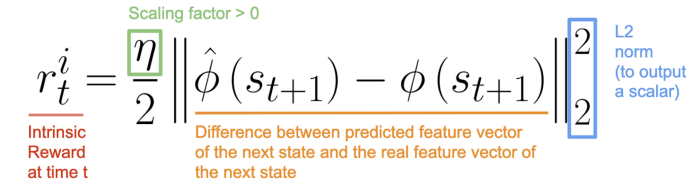Finally, the overall optimization problem of this module is a composition of Inverse Loss and Forward Loss.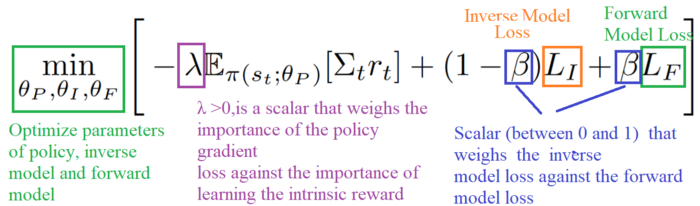And we provide the prediction error of the forward dynamics model to the agent as an intrinsic reward to encourage its curiosity.

Phew, that was a lot of information and mathematics! To recap:

• Because of extrinsic rewards implementation and sparse rewards problems, we want to create a reward that is intrinsic to the agent.
• To do that, we created curiosity, which is the agent’s error in predicting the consequence of its action given its current state.
• Using curiosity, we will push our agent to favor transitions with high prediction error (which will be higher in areas where the agent has spent less time, or in areas with complex dynamics) and consequently better explore our environment.
• Because we can’t predict the next state by predicting the next frame (it’s too complicated), we use a better feature representation that will keep only elements that can be controlled by our agent or affect our agent.
• To generate curiosity, we use the Intrinsic Curiosity module that is composed of two models: the Inverse Model, which is used to learn the feature representation of state and next state, and the Forward Dynamics model, used to generate the predicted feature representation of the next state.
• Curiosity will be equal to the difference between hat_Φ(s(t+1))(Forward Dynamics model) and Φ(s(t+1)) (Inverse Dynamics model).

## ICM With Super Mario Bros

We wanted to see if our agent was able to play Super Mario Bros using only curiosity as reward. In order to do that, we used the official implementation of Deepak Pathak et al. We trained our agent on 32 parallel environments for 15 hours on a small NVIDIA GeForce 940M​ GPU.

We can see in this video that our agent was able to beat the first level of Super Mario Bros without extrinsic rewards, which proves the fact that curiosity is a good reward to train our agent.